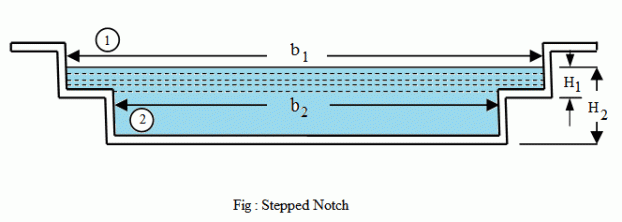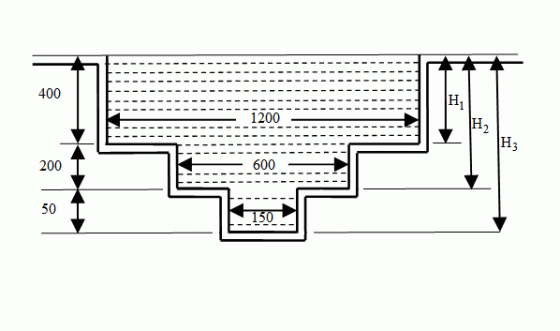•http://facebook.com/
•https://www.google.com/accounts/o8/id
•https://me.yahoo.com

# Stepped Notch

Discharge over a Stepped Notch

## Overview

A stepped notch is a combination of rectangular notches as shown in figure. It is thus obvious that the discharge over such a notch will be the sum of the discharges over the different rectangular notches.Consider a stepped notch as shown in figure. For the purpose of analysis, let us split up the notch into two rectangular notches 1 and 2. The total discharge over the notch will be the sum of the discharges over the two rectangular notches.

Let,
• H1 = Height of the liquid above the sill of notch 1
• b1 = Breadth of notch 1
• H2, b2 = Corresponding values for notch 2, and
• Cd = Coefficient of discharge for both the notches

From the geometry of the notch, we get,

and the discharge over the notch 2,

Now the total discharge over the notch,

Example:
[metric]
##### Example - Discharge over a Stepped Notch
Problem
Find the discharge in m3 /s over a stepped notch shown in figure.The level of water coincides with the top of the notch. Take Cd for all sections 0.6. All dimensions are in mm.
Workings
Given,
• Cd = 0.6

We know that the total discharge over the stepped notch,

Solution
Discharge over the notch = 0.779 m3 /s